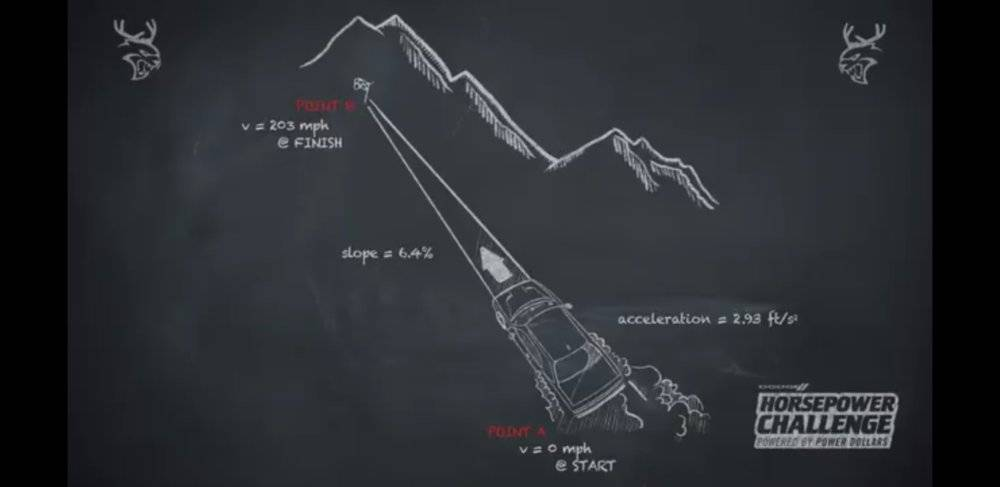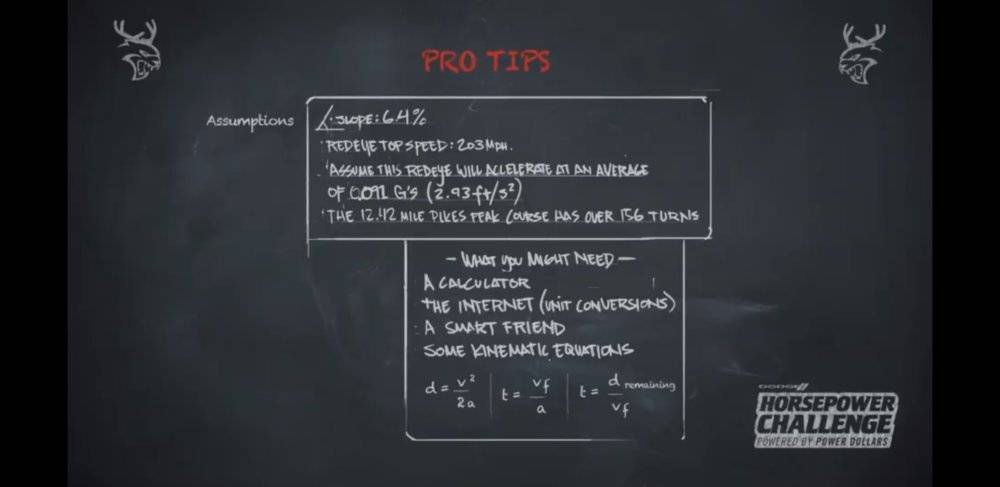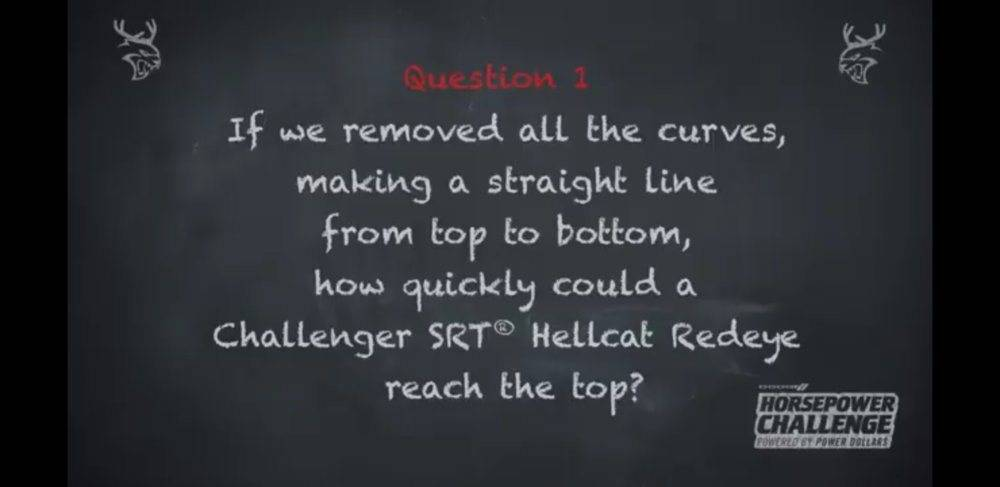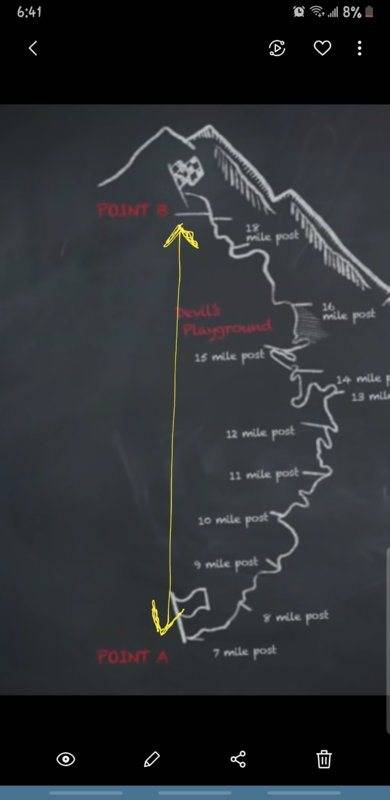# Car accelerating up a hill

• nateb27
This car will accelerate at an average of .091 g (2.93 ft/s2).The 12.42 mile long mountain course has over 156 turns. (Not sure what to do with this information. It says we remove all the turns, so the course should no longer be 12.42 miles, right?)GIVEN EQUATIONS:d=v^2/2at=v_f/at=d_remaining/v_fI got that the time is equal to 1 minute and 41 seconds on one attempt, another gave 3 minutes and 7 seconds, and my latest attempt gave 4 minutes and 1 second. If anyone can

#### nateb27

Homework Statement
Find the time that it takes for a car to reach the top of a hill.
The car can accelerate at 2.93 ft/s^2
The hill's slope = 6.4 %
The final velocity = 203mph
The initial velocity = 0mph
Relevant Equations
d = v^2/ 2a
t = v (f) / a
t = d (remaining) / v (f)
QUESTION:
If all the curves were removed, making a straight line from top to bottom, how quickly could a car reach the top of a mountain?

VARIABLES:
The mountain has a slope of 6.4%
The car's initial velocity is 0.
The car's top speed is 203 mph, (which will also be its final velocity as it crosses the finish line)
This car will accelerate at an average of .091 g (2.93 ft/s2).
The 12.42 mile long mountain course has over 156 turns. (Not sure what to do with this information. It says we remove all the turns, so the course should no longer be 12.42 miles, right?)

GIVEN EQUATIONS:
d=v^2/2a
t=v_f/a
t=d_remaining/v_f

I got that the time is equal to 1 minute and 41 seconds on one attempt, another gave 3 minutes and 7 seconds, and my latest attempt gave 4 minutes and 1 second. If anyone can help me get the answer, I would greatly appreciate it.

Welcome to the PF, Nate.Can you post your work for the 3 methods so we can look for mistakes? That would be the easiest way to try to help you figure it out. Thanks for making such a complete problem statement post, BTW.

You can use the "Attach files" button below the Edit window to upload a PDF or JPEG or similar image of your work. Please be sure the picture is clear, and right-side-up. Thanks.

nateb27 said:
Homework Statement: Find the time that it takes for a car to reach the top of a hill.
The car can accelerate at 2.93 ft/s^2
The hill's slope = 6.4 %
The final velocity = 203mph
The initial velocity = 0mph
Homework Equations: d = v^2/ 2a
t = v (f) / a
t = d (remaining) / v (f)

QUESTION:
If all the curves were removed, making a straight line from top to bottom, how quickly could a car reach the top of a mountain?

VARIABLES:
The mountain has a slope of 6.4%
The car's initial velocity is 0.
The car's top speed is 203 mph, (which will also be its final velocity as it crosses the finish line)
This car will accelerate at an average of .091 g (2.93 ft/s2).
The 12.42 mile long mountain course has over 156 turns. (Not sure what to do with this information. It says we remove all the turns, so the course should no longer be 12.42 miles, right?)

GIVEN EQUATIONS:
d=v^2/2a
t=v_f/a
t=d_remaining/v_f

I got that the time is equal to 1 minute and 41 seconds on one attempt, another gave 3 minutes and 7 seconds, and my latest attempt gave 4 minutes and 1 second. If anyone can help me get the answer, I would greatly appreciate it.
So much in the problem statement is unclear.
Is the quoted max acceleration when going up the straight line (6.4% incline) or on the flat? If the former, the slope information is irrelevant.
Are we supposed to assume the max acceleration is achieved all the way to the finish or only until it reaches max speed?
Why is the 2.93 ft/s2 first given as max acceleration then as "average"? Why not just say it is the acceleration?

•collinsmark
"haruspex" The acceleration is "average" going up the incline until Vf 203MPH

ojorojo said:
"haruspex" The acceleration is "average" going up the incline until Vf 203MPH
That doesn't address either of my quibbles.

It is also stated as a maximum ("can accelerate at..."). If the number is both average and maximum over an interval it must be constant.

It is still unclear whether, having accelerated up the max speed, it has reached the top of the hill or continues at that max speed for a way.

•scottdave
haruspex said:
That doesn't address either of my quibbles.

It is also stated as a maximum ("can accelerate at..."). If the number is both average and maximum over an interval it must be constant.

It is still unclear whether, having accelerated up the max speed, it has reached the top of the hill or continues at that max speed for a way.
It says the car WILL accelerate at that average speed..203mph is top speed

. Slope of 6.4%
. Car top speed 203 mph
. Car has a peak
acceleration of over I g. Assume
this car will accelerate at an
average of .091 g (2.93 ft/s2)
The 1 2.42 mile long Pike's Peak
course has over 156 turns

QUESTION:
If all the curves were removed, making a straight line from top to bottom, how quickly could a car reach the top of a mountain?

I'm assuming the 6.4% slope has to be factored in in order to get a "true" acceleration...so far I've gotten an angle of 3.66 degrees from that slope. now trying to factor in the "G's" effect from that slope into the given acceleration

nateb27 said:
The car's top speed is 203 mph, (which will also be its final velocity as it crosses the finish line)
This car will accelerate at an average of .091 g (2.93 ft/s2).
Since we are given average acceleration and top speed we can compute elapsed time. We can then divide total distance by that elapsed time to get average speed. The resulting average speed exceeds 203 miles per hour.

The problem statement is inconsistent.

still got to account for the slope and its G force impact on the car

@ojorojo -- are you related to the OP account @nateb27 ? Just curious. I got vertigo when the replies to questions meant for the OP started coming from you.••scottdave and phinds
Lol...I understand. I have the same problem as the OP is trying to solve that's all. But i don't see Nate chipping in so I'm assuming he is just reading the posts..Im not sure if this is the formula i should use to find the true acceleration
a=g*SIN(tetha)...would that be a negative? as in:
a=-9.8m/s2*(3.66)...?thanks you

•berkeman
ojorojo said:
a=g*SIN(tetha)...would that be a negative? as in:
a=-9.8m/s2*(3.66)...?thanks you
Not following that. How is ##\sin \theta## going to get as high as 3.66?

I have been working on this same question. Can you review the attached for any errors?

#### Attachments

•4ahHd.png
85.2 KB · Views: 541
jbriggs444 said:
We can then divide total distance by that elapsed time
The given distance is along the real, curvy road, not the fictitious straight line road. It is unclear whether, even with the straight line course, the max acceleration applies all the way to the top of the hill. In the curv d road case presumably it does not: the maintains the given acceleration up to the max speed then continues at that speed.
That said, it makes no sense that the same max acceleration would apply in both scenarios.

ojorojo said:
still got to account for the slope and its G force impact on the car
As I posted, that is unclear. See post #3. I.e. does the given max acceleration refer to driving on the flat or when driving up this hill?

•scottdave
ojorojo said:
It says the car WILL accelerate at that average speed..203mph is top speed
You mean at that average rate, yes? An acceleration is not a speed. And it says that acceleration is both the average and the max, which can only be true if it is constant.

But this still leaves open the possibility that it reaches the max speed before reaching the top of the hill, then continues at that max speed.
The text in post #1 suggests to me that the acceleration applies all the way to the top, which yields an easy way to find an answer. If that is not the intention, I do not think there is enough information. @nateb27 posted several answers, but we have yet to see how these were obtained.

ingenius1 said:
D = 12.42 miles.
That is along the real, curvy road, not the straight line we are being asked to consider.

Looks to me that start (a) to finish (b) are completely uphill and the speed is 0 at (a) to 203mph at (b)...yes 12.42 miles are the total real course mileage including 156 turns...the mileage to be ran its assumed to be shorter

ojorojo said:
Looks to me that start (a) to finish (b) are completely uphill and the speed is 0 at (a) to 203mph at (b)...
Yes, but that does still not specify whether it reaches top speed before the top of the hill. We are told the max (constant) acceleration applies until the max speed is reached, and that the max speed applies at the top, but not whether the acceleration applies to the top.how can one figure remaining distance on the given 12.42miles if its all turns and curves regardles of how many miles the car has traveled on a straight line?.its the 12.42m just there to mislead people on this calculation?

Unsolvable..?.Why so salty?..can u work with what's presented and not assume anything like oxigen levels and stuff like that but the given the assumed acceleration?

I think u are really misinterpreting the picture.
Pikes Peak its a real race course..and including its 156 turns its actually a total of 12.42m from start to finish. What its asked here is to eliminate the curves..GET OFF THE ACTUAL COURSE and pretend to drive straight through from start to finish..straight line..which at the end of the day it could be 7 to 9 miles total..(im just assuming).

What's mostly missing is the common sense step of verifying what the high school homework problem actually is, determining what elements should be included, and if there's any variables that are missing or need clarification.

•Tom.G
ojorojo said:
Thanks for posting the original exactly.
This suggests to me that the acceleration quoted is from some genuine data for the vehicle, hence is for the flat, and you do need to discount for the gradient.
However, the same view of the top speed number leads to the likelihood that the top speed is reached before the hilltop is attained. That would leave us with insufficient data to solve the problem.

ingenius1 said:
If we removed all the curves, making a straight line from top to bottom, how quickly could the car make it to the top?
Making it a straight line means it is a lot less than 12 miles. Shouting doesn't change that.
ingenius1 said:
you use the formulas to figure all of that out.
Without knowing the distance, how? You seem to be arguing it surely wouid reach top speed before hill top, but then we have insufficient data to answer the question.

ojorojo said:
12.42 miles are the total real course mileage including 156 turns...the mileage to be ran its assumed to be shorter
I offer an alternative assumption, that the curves are large enough radius that full speed can be maintained around them. (Since there was no map included, that still fits the description.)

Tom.G said:
I offer an alternative assumption, that the curves are large enough radius that full speed can be maintained around them. (Since there was no map included, that still fits the description.)
I do not see the relevance of that observation. The curvy course of 12.42 miles is mere background and of no significance in the question being asked.

•ingenius1
ingenius1 said:
then i guess you can't answer that problem then, can you?

•Could we assume that in this case 203mph is reached at the moment the car crosses the finish line and consider it Vf..? Could the time from "a" to "b" be determined then...?Theres Pikes Peak. No curves..forget curves...straight shot from point A to point B...with an incline of 6.4% thrown in that line

BTW..fastest time ever ran is 7:57..That's throught the whole 12.42miles...Just FYI if curious...nuthin to do with the problem...The problem statement, as written, has a few ambiguities in it. The main one regards what is meant by "if we removed all the curves ... ."

Interpretation A:
Keep the 3-dimensional starting location and finish location exactly where they are, and then draw a new, straight line between them, and that is the new track.

However, this will increase the slope above 6.4 %, if that even matters.

Without knowing more information the relative position between the starting location and finish location, there isn't enough information to solve this problem, in this interpretation.

Interpretation B:
Grab the starting position and pull it out horizontally until the track becomes a taught, straight line. In this interpretation, the length of the track is still 12.42 miles and the slope is still 6.4% (if the slope even matters).

Other ambiguities:
The maximum acceleration is specified as $2.93 \ \mathrm{\frac{ft}{s^2}}$. But is this the maximum acceleration with respect to the road when climbing a 6.4% slope, or $2.93 \ \mathrm{\frac{ft}{s^2}}$ total?

You see, initially when the vehicle is accelerating, it will be fighting the acceleration of gravity somewhat due to the fact that it's on a slope. Should this be taken into account, or is it already taken into account in the $2.93 \mathrm{\frac{ft}{s^2}}$ specification?

Also, I looked up the Pikes Peak International Hill Climb. Its track length is 12.42 Miles with an elevation change (from start to finish) of 4725 ft. That produces an average slope gradient of 7.22%, although this problem says to use only 6.4%, and honestly, I'm not certain whether the slope is relevant to solving this problem at all, given the other ambiguities.

Last edited: